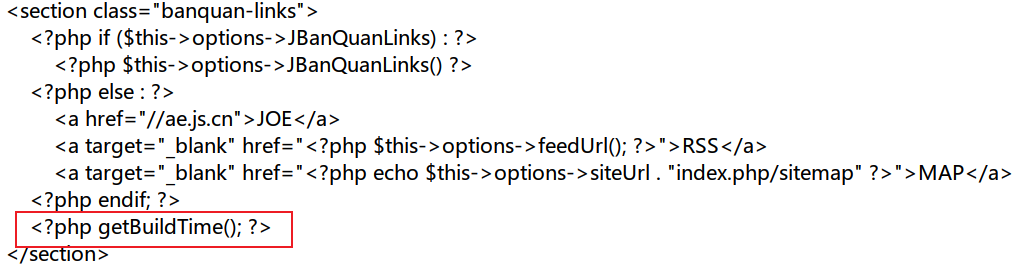• 累计撰写 80 篇文章
• 累计收到 145 条评论

# 在网站的下方添加站点运行时间2021-03-23 / 0 评论 / 1,863 阅读 / 674 字数 / 正在检测是否收录...

## 在网站的下方添加站点运行时间

2021.07.03 - 更新：

### 1.修改function.php文件

// 设置时区
date_default_timezone_set('Asia/Shanghai');
/**
* 秒转时间，格式 年 月 日 时 分 秒
*
*/
function getBuildTime() {
// 在下面按格式输入本站创建的时间
$site_create_time = strtotime('2020-11-29 00:00:00');$time = time() - $site_create_time; if (is_numeric($time)) {
$value = array( "years" => 0, "days" => 0, "hours" => 0, "minutes" => 0, "seconds" => 0, ); if ($time >= 31556926) {
$value["years"] = floor($time / 31556926);
$time = ($time % 31556926);
}
if ($time >= 86400) {$value["days"] = floor($time / 86400);$time = ($time % 86400); } if ($time >= 3600) {
$value["hours"] = floor($time / 3600);
$time = ($time % 3600);
}
if ($time >= 60) {$value["minutes"] = floor($time / 60);$time = ($time % 60); }$value["seconds"] = floor($time); //在下面可以修改站点运行时间的文字说明和颜色等 echo '本站点运行了<span class="btime" style="color:#6495ED">'.$value['years'].
'年'.$value['days']. '天'.$value['hours'].
'小时'.\$value['minutes'].
'分</span>';
} else {
echo '';
}
}

### 2.修改footer.php文件

<?php getBuildTime(); ?>0